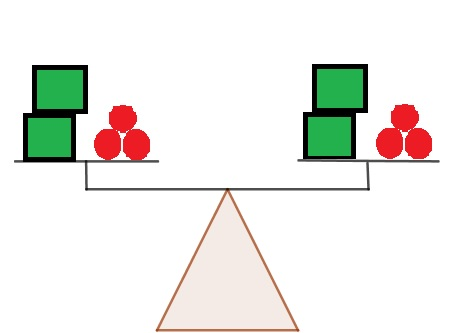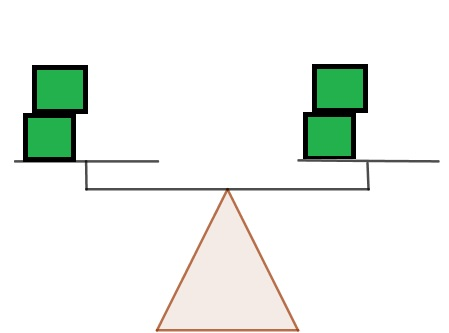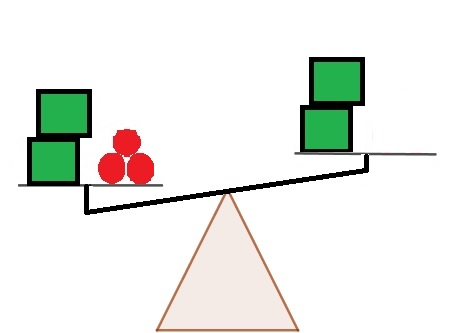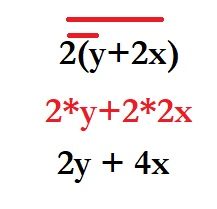Math Courses / Course / Chapter

# Simplifying and Solving Equivalent Equations: What are Equivalent Equations?

• Author

Joao Amadeu has more than 10 years of experience in teaching physics and mathematics at different educational levels. Joao earned two degrees at Londrina State University: B.S. in Physics and M.S. in Science and Mathematics Education. He is currently working on his PhD in Science Education at Western Michigan University.

• Instructor
Michelle Vannoy

Michelle holds a M.S. in Science Education and a B.S. in Elementary Education. She has extensive teaching experience, and has taught middle school maths and sciences, as well as a variety of other subjects as an interim teacher.

This lesson focuses on describing equivalent equations. It shows how to solve them through solved problems and examples. Updated: 12/10/2021

Show

#### How do you find equivalent equations?

Equivalent equations are ones the give the same values for their variables. One equation can be simplified to the point of finding one that is equivalent to it.

#### How do you show equivalent equations?

Equivalent equations can be shown in two main ways.

1 - Find that both yell the same values for their variables.

2-Simplify one of them until it looks similar to the other one.

#### What is an example of an equivalent equation?

x+7=10 and 2x+14=20

If the second equation is divided by two, it will be equivalent to the first one.

(2x+14)/2=20/2

x+7=10

#### What is the difference between equivalent expressions and equivalent equations?

This difference relies on the fundamental differences between equations and expressions. Equations have two parts divided by an equal sign, two equivalent equations will maintain their equivalency. Expressions are ideas expressed in algebraic language. Two expressions that express the same idea are equivalent.

Equivalent equations can be simplified before solving. In basic terms, equality will remain the same if whatever operation is made on one side of the equal sign is made also on the other sign of the equal sign.

Returning to the two examples given in the previous section, the equations 2x = 4 and 4x = 8 are equivalent. Therefore, one of the equations can be simplified to appear as the other one. It is easy to see that all the terms of 4x = 8 are twice the value of the terms of 2x = 4.

To unlock this lesson you must be a Study.com Member.

## Equivalent Equations

You are planning a family reunion and have decided to buy custom-printed shirts for everyone to wear. Two companies have been equally recommended by your friends. The first company, Too Cool Tees, charges $6 per shirt and$12 processing and handling fee. The second company, T-Shirts For Less, costs $7.50 per shirt and$9 processing and handling fee. You are not sure how many people are going to be at the family reunion. You ask yourself, at what point will the cost be the same from either company? Equivalent equations will answer this question.

Equivalent equations are two equations that have the same solution. They are used anytime multiple equations with the same variable need to equal each other, just like in our example.An error occurred trying to load this video.

Try refreshing the page, or contact customer support.

Coming up next: Solving Equations Using the Addition Principle

### You're on a roll. Keep up the good work!

Replay
Your next lesson will play in 10 seconds
• 0:04 Equivalent Equations
• 0:50 Setting Up To Solve
• 1:43 Solving
• 4:02 Uses
• 4:43 Lesson Summary
Save Timeline
Autoplay
Autoplay
Speed Speed

An equation is considered to be solved when the values for any variables of the equation are found. An exception is when these values cannot be found only through the equation; in this case, the equation can be considered solved if it is fully simplified. When comparing two equations for equivalency, one of the equations must be simplified until it obtains (or not) the same terms as the other one. Solving equivalent equations can be useful to find a point where equations are equal and variables have the same value, as well as to find break-even points.

Considering the equation -4x + 12y + 100 = 0. All terms can be divided by 4 without producing fractions or decimals, therefore:

{eq}\frac {-4x}{4} + \frac {12y}{4} + \frac {100}{4} = \frac {0}{4} {/eq}

-x + 3y + 25 = 0

The equations -4x + 12y + 100 = 0 and -x + 3y + 25 = 0 are equivalent.

One can notice that the simplified version of the equation allows one to better observe, or even isolate, the variables. For example, one can add x to both sides of the equation and isolate this variable.

(+x) -x + 3y + 25 = 0 (+x)

3y + 25 = x

To unlock this lesson you must be a Study.com Member.

An equation can be seen as two sides of a scale. If the equality is true, then there is a balance between both sides as shown in Figure 1.If both sides of the scale lose an equal piece, the scale remains balanced.If only one side of the scale loses a piece, then the balance is gone.That works in equations solution in the sense of, for the equal sign to remain valid, both sides need to be operated in the same way (e.g.: divided by 2).

Equivalent equations are different than equivalent expressions since the latter ones do not present equal signs. An example of equal expressions is 2y + 4x and 2(y + 2x). If one applies multiplication to the second equation, it becomes exactly like the first one.### Equivalent Equations Examples

Example 1
Test if the equations from items a to c are equivalent to 4x+3=2x-3.

a) 8x+6=4x-3

b) 4x=2x-6

c) 4x+4=2x-2

Solution

a) 8x+6=4x-3

Dividing the equation by 2:

4x+3=2x-1.5

It is not equivalent to the original equation as per the value of the last constant.

b) 4x=2x-6

Adding 3 to both sides of the equation:

4x+3=2x-3

The equations are equivalent.

c) 4x+4=2x-2

To unlock this lesson you must be a Study.com Member.

This lesson described equivalent equations. These were explained as being equations that produce the same roots, resulting in equal values or equal equations after simplification. An equation can be simplified by performing operations such as addition, subtraction, division, and multiplication on both sides of the equation. For example, the equation 4x-6y=8 can have both sides divided by two. Therefore, it will be equivalent to the equation 2x-3y=4. The terms can still be rearranged and form a third equivalent equation, for example, 2x-3y-4=0.

To unlock this lesson you must be a Study.com Member.

## Setting Up to Solve

Let x equal the number of t-shirts ordered.

The equation for each of the two companies for the cost of the shirt plus processing and handling fees would be:

Too Cool Tees: 6x + 12 = total shirts bought

T-Shirts For Less: 7.50x +9 = total shirts bought

Since you do not know how many family members are coming to the reunion yet and you want to see at what point the t-shirts from the two companies would cost the same, you would set the equations equal to each other and solve. Anytime a problem tells you to find when two equations are the same, this means to set the two equations equal to each other. Your problem for our story would look like this: 6x + 12 = 7.50x +9. Solving for x tells you how many shirts would cost the same amount, no matter which company you order from.

## Solving

To solve any multi-step equation, you need to do a set of five steps in order. Not all problems will have all steps present, but you should check for them in order anyways.

### Step 1

Check for the distributive property on each side of the equal sign. If present, complete the distributive property. There is no distributive property in our example.

### Step 2

Check for combining like terms on each side of the equal sign. If present, combine all like terms on the same sign of the equal sign. There are no like terms present in our example.

### Step 3

Collect all the variables on the same side of the equal sign. Do this by adding or subtracting the variable with its coefficient from both sides of the equation. Add or subtract based on whichever is opposite of the current operation. By subtracting 7.50x (subtracting is opposite of a positive 7.50x), we can move the x's on the same side of the equation. What you do to one side of the equation you must do to the other side to keep the equation equal.

### Step 4

Collect all constants on the opposite side of the equation as the variable. You can do this by adding or subtracting the constant from both sides of the equation. Again, add or subtract based on whichever is the opposite of the current operation. The 12 is a positive 12 so we do the opposite operation, which would be subtracting 12.

Video Transcript

## Equivalent Equations

You are planning a family reunion and have decided to buy custom-printed shirts for everyone to wear. Two companies have been equally recommended by your friends. The first company, Too Cool Tees, charges $6 per shirt and$12 processing and handling fee. The second company, T-Shirts For Less, costs $7.50 per shirt and$9 processing and handling fee. You are not sure how many people are going to be at the family reunion. You ask yourself, at what point will the cost be the same from either company? Equivalent equations will answer this question.

Equivalent equations are two equations that have the same solution. They are used anytime multiple equations with the same variable need to equal each other, just like in our example.

## Setting Up to Solve

Let x equal the number of t-shirts ordered.

The equation for each of the two companies for the cost of the shirt plus processing and handling fees would be:

Too Cool Tees: 6x + 12 = total shirts bought

T-Shirts For Less: 7.50x +9 = total shirts bought

Since you do not know how many family members are coming to the reunion yet and you want to see at what point the t-shirts from the two companies would cost the same, you would set the equations equal to each other and solve. Anytime a problem tells you to find when two equations are the same, this means to set the two equations equal to each other. Your problem for our story would look like this: 6x + 12 = 7.50x +9. Solving for x tells you how many shirts would cost the same amount, no matter which company you order from.

## Solving

To solve any multi-step equation, you need to do a set of five steps in order. Not all problems will have all steps present, but you should check for them in order anyways.

### Step 1

Check for the distributive property on each side of the equal sign. If present, complete the distributive property. There is no distributive property in our example.

### Step 2

Check for combining like terms on each side of the equal sign. If present, combine all like terms on the same sign of the equal sign. There are no like terms present in our example.

### Step 3

Collect all the variables on the same side of the equal sign. Do this by adding or subtracting the variable with its coefficient from both sides of the equation. Add or subtract based on whichever is opposite of the current operation. By subtracting 7.50x (subtracting is opposite of a positive 7.50x), we can move the x's on the same side of the equation. What you do to one side of the equation you must do to the other side to keep the equation equal.

### Step 4

Collect all constants on the opposite side of the equation as the variable. You can do this by adding or subtracting the constant from both sides of the equation. Again, add or subtract based on whichever is the opposite of the current operation. The 12 is a positive 12 so we do the opposite operation, which would be subtracting 12.

To unlock this lesson you must be a Study.com Member.

### Register to view this lesson

Are you a student or a teacher?

Back

### Resources created by teachers for teachers

Over 30,000 video lessons & teaching resources‐all in one place.Video lessonsQuizzes & WorksheetsClassroom IntegrationLesson Plans

I would definitely recommend Study.com to my colleagues. It’s like a teacher waved a magic wand and did the work for me. I feel like it’s a lifeline.

Jennifer B.
TeacherCreate an account to start this course today
Used by over 30 million students worldwide